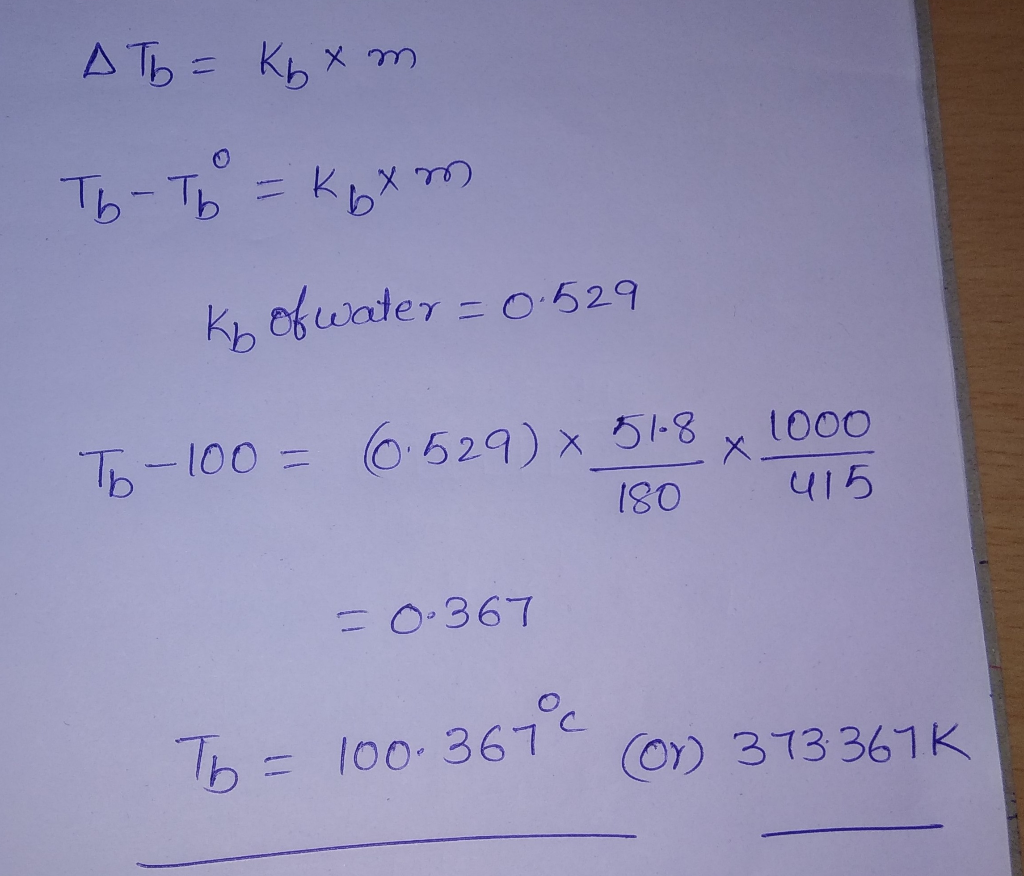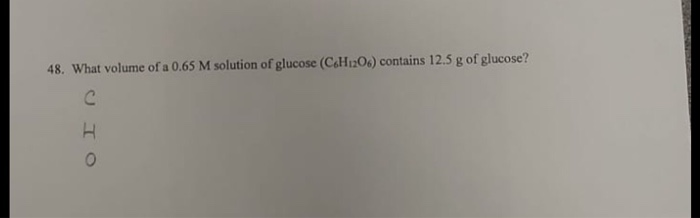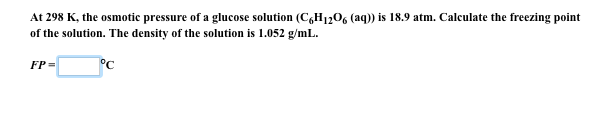Question

# a glucose solution contains 51.8 glucose (C6H12O6) in 415 g of water calculate the boiling point...

a glucose solution contains 51.8 glucose (C6H12O6) in 415 g of water

calculate the boiling point of the solution#### Earn Coins

Coins can be redeemed for fabulous gifts.

Similar Homework Help Questions
• ### A glucose solution contains 51.8 g of glucose (C6H12O6) in 415 g of water. Calculate the...

A glucose solution contains 51.8 g of glucose (C6H12O6) in 415 g of water. Calculate the freezing point of the solution. (Assume a density of 1.00 g/mL for water.)

• ### What is the freezing point of a solution that contains 12.0 g of glucose (C6H12O6) in 50.0 g of...

What is the freezing point of a solution that contains 12.0 g of glucose (C6H12O6) in 50.0 g of water (Kf = 1.86°C/m)a. -2.48 °Cb.-2.00 °Cc.-0.682 °Cd.-0.124 °C

• ### 1. What mass of 40.0% glucose, C6H12O6, solution contains 75.0 g of water?

1. What mass of 40.0% glucose, C6H12O6, solution contains 75.0 g of water?

• ### Determine the vapor pressure of a solution at 25°C that contains 76.6 g of glucose (C6H12O6) in 250.0 mL of water. The...

Determine the vapor pressure of a solution at 25°C that contains 76.6 g of glucose (C6H12O6) in 250.0 mL of water. The vapor pressure of pure water at 25°C is 23.8 torr. Please show work...

• ### 48. What volume of a 0.65 M solution of glucose (C6H12O6) contains 12.5 g of glucose?48. What volume of a 0.65 M solution of glucose (C6H12O6) contains 12.5 g of glucose?

• ### A solution is prepared by dissolving 29.2 g of glucose (C6H12O6) in 355 g of water....

A solution is prepared by dissolving 29.2 g of glucose (C6H12O6) in 355 g of water. The final volume of the solution is 376 mL . For this solution, calculate each of the following. A.) molarity B.) moality C. ) percent by mass D.) mole fraction E.) mole percent

• ### Calculate the molality of a glucose solution, C6H12O6 (molar mass=180.2 g/mol) that is prepared by mixing...

Calculate the molality of a glucose solution, C6H12O6 (molar mass=180.2 g/mol) that is prepared by mixing 234 g of glucose in 755 g water

• ### Calculate the freezing point of a solution that contains 5.99 g of glucose, a non electrolyte,...

Calculate the freezing point of a solution that contains 5.99 g of glucose, a non electrolyte, dissolved in 101 g of water?

• ### At 298 K, the osmotic pressure of a glucose solution (C6H12O6 (aq)) is 18.9 atm. Calculate...At 298 K, the osmotic pressure of a glucose solution (C6H12O6 (aq)) is 18.9 atm. Calculate the freezing point of the solution. The density of the solution is 1.052 g/mL FP = oC

• ### What mass of glucose (C6H12O6) is needed to prepare 0.225m C6H12O6 using 250.g g of water?

What mass of glucose (C6H12O6) is needed to prepare 0.225m C6H12O6 using 250.g g of water?